# 图解Focal Loss以及Tensorflow实现（二分类、多分类）• 横轴是ground truth类别对应的概率（经过sigmoid/softmax处理过的logits），纵轴是对应的loss值；
• 蓝色的线（gamma=0），就是原始交叉熵损失函数，可以明显看出ground truth的概率越大，loss越小，符合常识；
• 除了蓝色的线，其他几个都是Focal Loss的线，其实原始交叉熵损失函数是Focal Loss的特殊版本（gamma=0）
• 其他几个Focal Loss线都在蓝色下边，可以看出Focal Loss的作用就是【衰减】；
• 从图中可以看出，ground truth的概率越大（即容易分类的简单样本），衰减越厉害，也就是大大降低了简单样本的loss；
• 从图中可以看出，ground truth的概率越小（即不易分类的困难样本），也是有衰减的，但是衰减的程度比较小；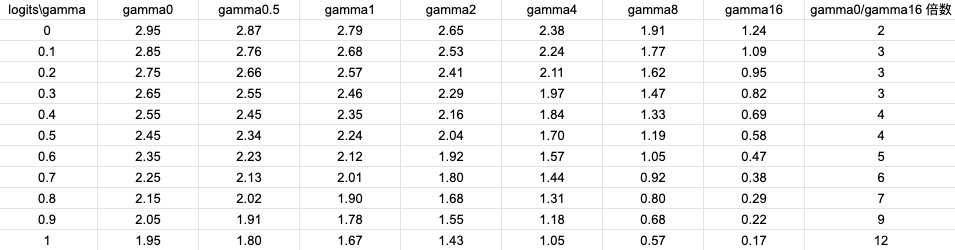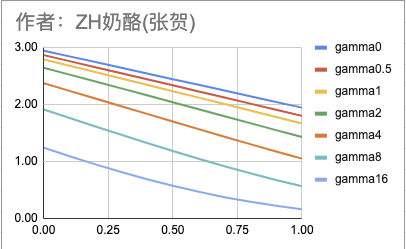• 当gamma为0的时候，等同于原始交叉熵损失；
• 二分类版本需要同时考虑正负样本的影响，多分类版本只需要考虑true label的影响，因为softmax的时候，已经考虑了其他labels；
• 多分类版本因为每个样本其实只需要1个值（即y_true one-hot向量中值为1的那个），所以有些实现会用tf.gather简化计算；

# 二分类Focal Loss

## 二分类交叉熵损失函数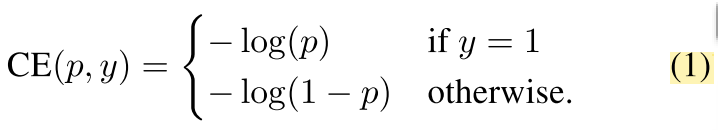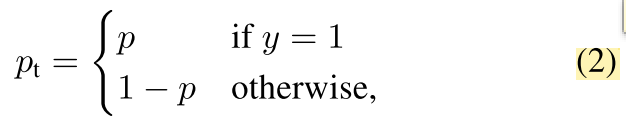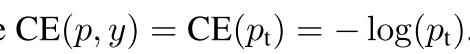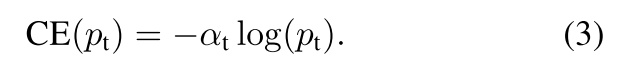## 二分类Focal Loss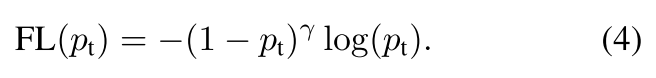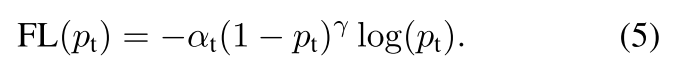## 二分类Focal Loss的Tensorflow实现

1. 要知道公式中的pt是类别对应的probs，而不是logits（logits经过sigmoid/softmax变成probs）；
2. 很多代码中都用y_pred变量，自己要搞清楚y_pred是指logits还是probs；
3. 二分类的p_t是要同时计算正/负样本的，这里和多分类有区别；

def binary_focal_loss(gamma=2, alpha=0.25):
alpha = tf.constant(alpha, dtype=tf.float32)
gamma = tf.constant(gamma, dtype=tf.float32)
def binary_focal_loss_fixed(n_classes, logits, true_label):
epsilon = 1.e-8
# 得到y_true和y_pred
y_true = tf.one_hot(true_label, n_classes)
probs = tf.nn.sigmoid(logits)
y_pred = tf.clip_by_value(probs, epsilon, 1. - epsilon)
# 得到调节因子weight和alpha
## 先得到y_true和1-y_true的概率【这里是正负样本的概率都要计算哦！】
p_t = y_true * y_pred \
+ (tf.ones_like(y_true) - y_true) * (tf.ones_like(y_true) - y_pred)
## 然后通过p_t和gamma得到weight
weight = tf.pow((tf.ones_like(y_true) - p_t), gamma)
## 再得到alpha，y_true的是alpha，那么1-y_true的是1-alpha
alpha_t = y_true * alpha + (tf.ones_like(y_true) - y_true) * (1 - alpha)
# 最后就是论文中的公式，相当于：- alpha * (1-p_t)^gamma * log(p_t)
focal_loss = - alpha_t * weight * tf.log(p_t)
return tf.reduce_mean(focal_loss)


# 多分类Focal Loss

## 多分类交叉熵损失函数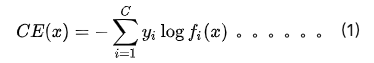## 多分类Focal Loss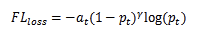## 多分类Focal Loss的Tensorflow实现

def test_softmax_cross_entropy_with_logits(n_classes, logits, true_label):
epsilon = 1.e-8
# 得到y_true和y_pred
y_true = tf.one_hot(true_label, n_classes)
softmax_prob = tf.nn.softmax(logits)
y_pred = tf.clip_by_value(softmax_prob, epsilon, 1. - epsilon)
# 得到交叉熵，其中的“-”符号可以放在好几个地方，都是等效的，最后取mean是为了兼容batch训练的情况。
cross_entropy = tf.reduce_mean(-tf.reduce_sum(y_true*tf.log(y_pred)))
return cross_entropy


def test_softmax_focal_ce_3(n_classes, gamma, alpha, logits, label):
epsilon = 1.e-8
# y_true and y_pred
y_true = tf.one_hot(label, n_classes)
probs = tf.nn.softmax(logits)
y_pred = tf.clip_by_value(probs, epsilon, 1. - epsilon)

# weight term and alpha term【因为y_true是只有1个元素为1其他元素为0的one-hot向量，所以对于每个样本，只有y_true位置为1的对应类别才有weight，其他都是0】这也是为什么网上有的版本会用到tf.gather函数，这个函数的作用就是只把有用的这个数取出来，可以省略一些0相关的运算。
weight = tf.multiply(y_true, tf.pow(tf.subtract(1., y_pred), gamma))
if alpha != 0.0:  # 我这实现中的alpha只是起到了调节loss倍数的作用（调节倍数对训练没影响，因为loss的梯度才是影响训练的关键），要想起到调节类别不均衡的作用，要替换成数组，数组长度和类别总数相同，每个元素表示对应类别的权重。另外[这篇](https://blog.csdn.net/Umi_you/article/details/80982190)博客也提到了，alpha在多分类Focal loss中没作用，也就是只能调节整体loss倍数，不过如果换成数组形式的话，其实是可以达到缓解类别不均衡问题的目的。
alpha_t = y_true * alpha + (tf.ones_like(y_true) - y_true) * (1 - alpha)
else:
alpha_t = tf.ones_like(y_true)

# origin x ent，这里计算原始的交叉熵损失
xent = tf.multiply(y_true, -tf.log(y_pred))

# focal x ent，对交叉熵损失进行调节，“-”号放在上一行代码了，所以这里不需要再写“-”了。
focal_xent = tf.multiply(alpha_t, tf.multiply(weight, xent))

# in this situation, reduce_max is equal to reduce_sum，因为经过y_true选择后，每个样本只保留了true label对应的交叉熵损失，所以使用max和使用sum是同等作用的。
reduced_fl = tf.reduce_max(focal_xent, axis=1)
return tf.reduce_mean(reduced_fl)


posted @ 2020-08-17 18:27  ZH奶酪  阅读(243)  评论(0编辑  收藏